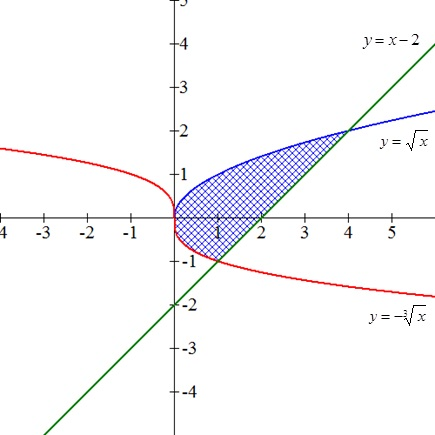Find area enclosed by y = square root x, y= - square root  {x}, y=x-2

Question:

Find area enclosed by

{eq}y= \sqrt x, \ \ y= - \sqrt  {x}, \ \ y=x-2 {/eq}

Definite integral as area of a region:

If {eq}\displaystyle f {/eq} is continuous and not negative in the closed interval {eq}\displaystyle [a,b] {/eq}, then the area of the region bounded by the graph of {eq}\displaystyle f {/eq}, the {eq}\displaystyle x {/eq}-axis and the vertical lines {eq}\displaystyle x=a {/eq} and {eq}\displaystyle x=b {/eq} is given by

{eq}\displaystyle A=\int_a^bf(x)dx {/eq}

For two curves:

{eq}\displaystyle A=\int_a^b[f(x)-g(x)]dx {/eq}

Become a Study.com member to unlock this answer! Create your account

The region is shown below:The area is calculated using the formula {eq}\displaystyle A=\int_a^b[f(x)-g(x)]dx {/eq}.

in this case, the area...The Fundamental Theorem of Calculus

from

Chapter 12 / Lesson 10
7.4K

The fundamental theorem of calculus is one of the most important points to understand in mathematics. Learn to define the formula of the fundamental theorem of calculus and explore examples of it put into practice.